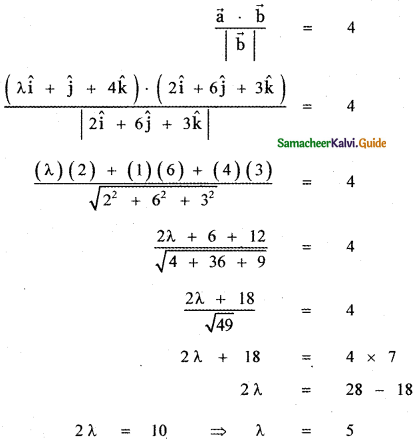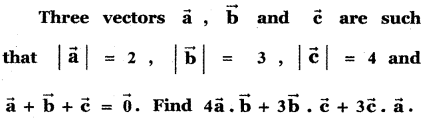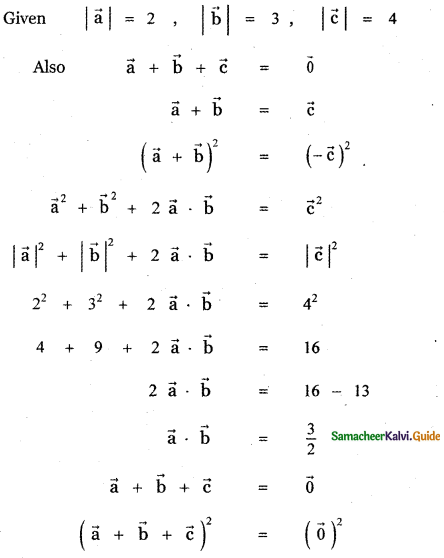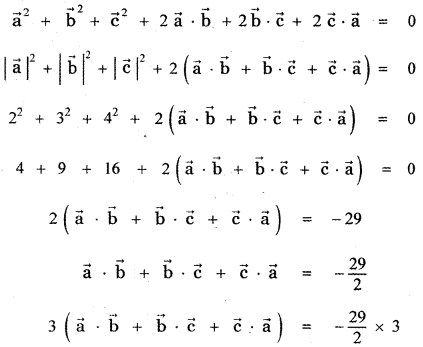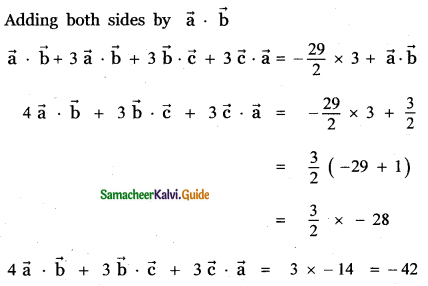Tamilnadu State Board New Syllabus Samacheer Kalvi 11th Maths Guide Pdf Chapter 8 Vector Algebra – I Ex 8.3 Text Book Back Questions and Answers, Notes.

## Tamilnadu Samacheer Kalvi 11th Maths Solutions Chapter 8 Vector Algebra – I Ex 8.3

Question 1.
Find $$\vec{a}$$ . $$\vec{b}$$ when
(i) $$\vec{a}$$ = î – 2ĵ + k̂ and $$\vec{b}$$ = 3î – 4ĵ – 2k̂
(ii) $$\vec{a}$$ = 2î + 2ĵ – k̂ and $$\vec{b}$$ = 6î – 3ĵ + 2k̂
(i) $$\vec{a}$$ = î – 2ĵ + k̂ and $$\vec{b}$$ = 3î – 4ĵ – 2k̂
$$\vec{a}$$ . $$\vec{b}$$ = (î – 2ĵ + k̂) . (3î – 4ĵ – 2k̂)
= (1) (3) + (-2) (-4) + (1) (-2)
= 3 + 8 – 2
= 9(ii) $$\vec{a}$$ = 2î + 2ĵ – k̂ and $$\vec{b}$$ = 6î – 3ĵ + 2k̂
$$\vec{a}$$ . $$\vec{b}$$ = (2î + 2ĵ – k̂) . (6î – 3ĵ + 2k̂)
= (2) (6) + (2) (-3) + (-1) (2)
= 12 – 6 – 2 = 12 – 8 = 4

Question 2.
Find the value λ for which the vectors $$\vec{a}$$ and $$\vec{b}$$ are perpendicular, where
(i) $$\vec{a}$$ = 2î + λĵ – k̂ and $$\vec{b}$$ = î – 2ĵ + 3k̂
(ii) $$\vec{a}$$ = 2î + 4ĵ – k̂ and $$\vec{b}$$ = 3î – 2ĵ + λk̂
When $$\vec{a}$$ and $$\vec{b}$$ are ⊥r then $$\vec{a} \cdot \vec{b}$$ = 0
$$\vec{a}$$ ⊥r $$\vec{b}$$ ⇒ $$\vec{a} \cdot \vec{b}$$ = 0
(i) (2) (1) + (λ) (-2) + (1) (3) = 0 ⇒ λ = 5/2
(ii) (2) (3) + (4) (-2) + (-1) (λ) = 0
6 – 8 – λ = 0
-λ – 2 = 0 ⇒ -λ = 2 ⇒ λ = -2Question 3.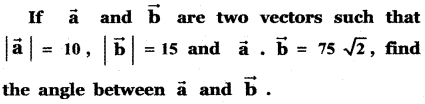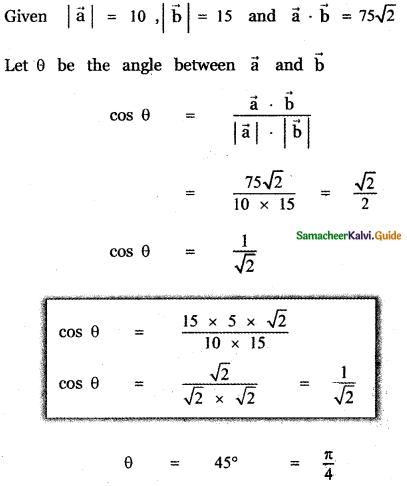Question 4.
Find the angle between the vectors
(i) 2î + 3ĵ – 6k̂ and 6î – 3ĵ + 2k̂
(ii) î – ĵ and ĵ – k̂
(i) 2î + 3ĵ – 6k̂ and 6î – 3ĵ + 2k̂
Let θ be the angle between the given vectors, then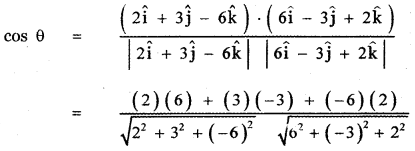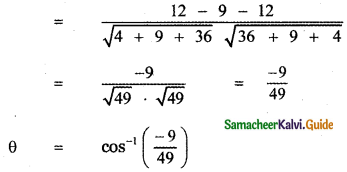(ii) î – ĵ and ĵ – k̂
Let θ be the angle between the given vectors, then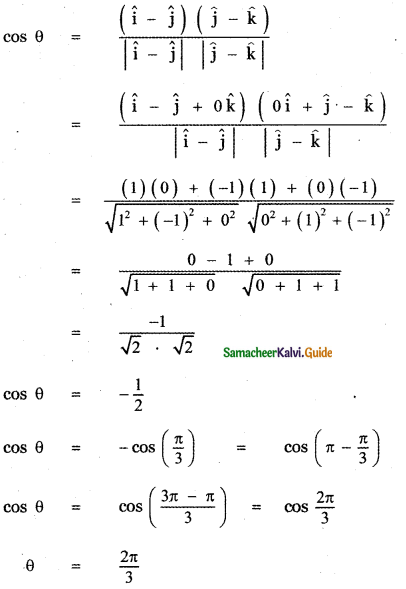Question 5.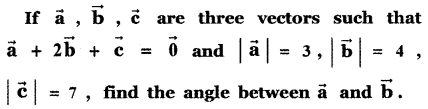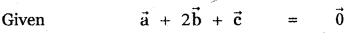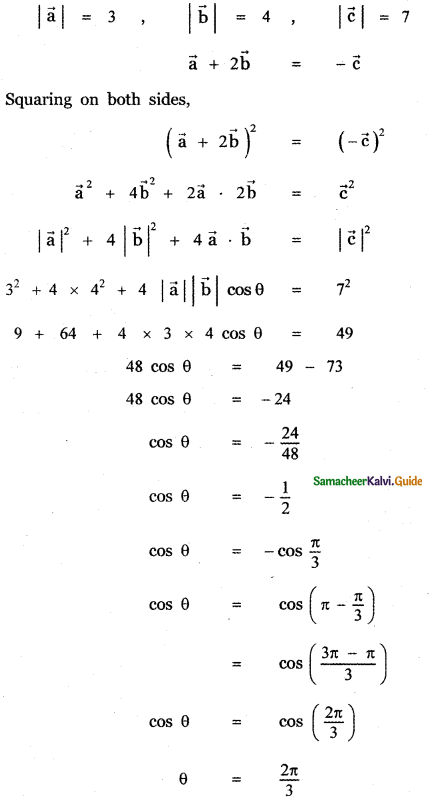Question 6.
Show that the vectors $$\vec{a}$$ = 2î + 3ĵ + 6k̂ $$\vec{b}$$ = 6î + 2ĵ – 3k̂ and $$\vec{c}$$ = 3î – 6ĵ + 6k̂ are mutually orthogonal.
Given $$\vec{a}$$ = 2î + 3ĵ + 6k̂ $$\vec{b}$$ = 6î + 2ĵ – 3k̂ and $$\vec{c}$$ = 3î – 6ĵ + 6k̂
$$\vec{a}$$ . $$\vec{b}$$ = (2î + 3ĵ + 6k̂) . (6î + 2ĵ – 3k̂)
= (2) (6) + (3) (2) + (6) (-3)
= 12 + 6 – 18
= 0
∴ $$\vec{a}$$ and $$\vec{a}$$ are perpendicular.

$$\vec{b}$$ . $$\vec{c}$$ = (6î + 2ĵ – 3k̂) . (3î – 6ĵ + 6k̂)
= (6) (3) + (2) (- 6) + (-3) (2)
= 18 – 12 – 6
= 0
∴ $$\vec{b}$$ and $$\vec{c}$$ are perpendicular.

$$\vec{c}$$ . $$\vec{a}$$ = (3î – 6ĵ + 6k̂) . (2î + 3ĵ + 6k̂)
= (3) (2) + (-6) (3) + (2) (6)
= 6 – 18 + 12
= 0
∴ $$\vec{c}$$ and $$\vec{a}$$ are perpendicular.
Hence $$\vec{a}$$, $$\vec{b}$$, $$\vec{c}$$ are mutually perpendicular vectors.

Question 7.
Show that the vectors – î – 2ĵ – 6k̂, 2î – ĵ + k̂ and – î + 3ĵ + 5k̂ from a right-angled triangle.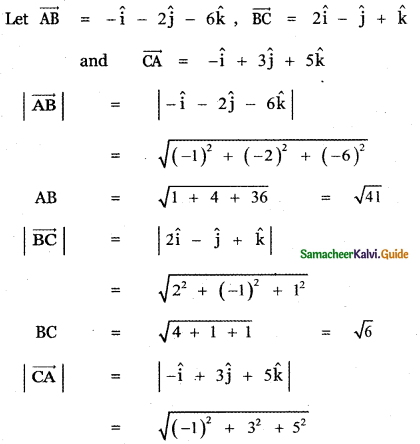CA = $$\sqrt{1+9+25}$$ = $$\sqrt{35}$$
AB ≠ BC + CA
∴ The given vectors form a triangle, Also
AB2 = 41, BC2 = 6, CA2 = 35
AB2 = BC2 + CA2
∴ ∆ ABC is a right angled triangle.Question 8.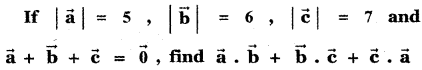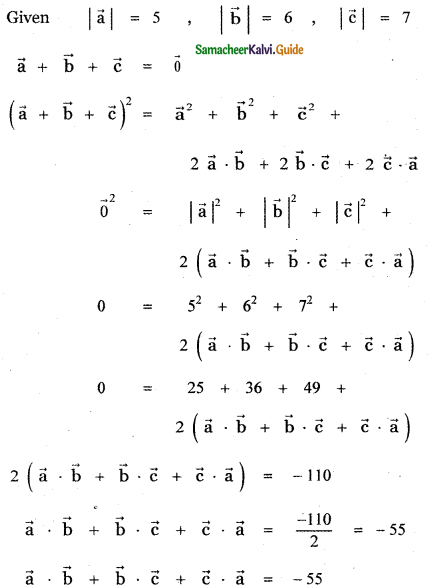Question 9.
Show that the points (2, – 1, 3), (4, 3, 1), and (3, 1, 2) are collinear.
Let the given points be
A (2, -1, 3), B (4, 3, 1) and C (3, 1, 2)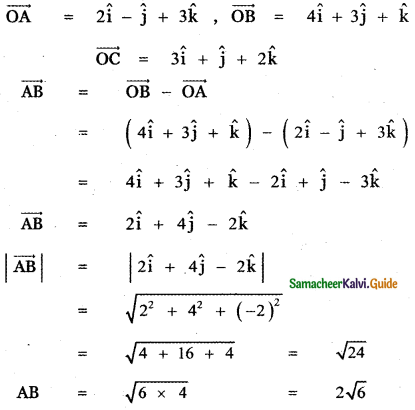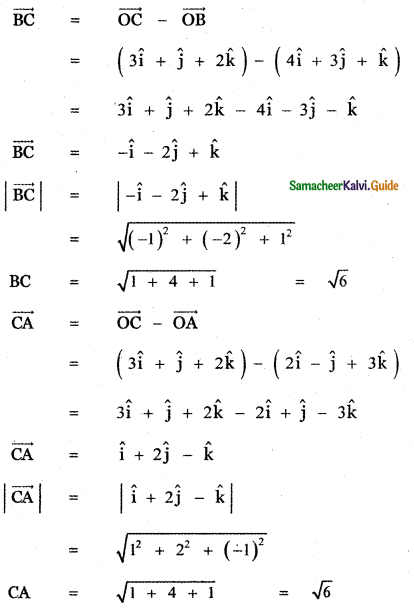AB = 2√6, BC = √6, CA = √6
BC + CA = √6 + √6 = 2√6
∴ BC + CA = BA = 2√6
Hence the given points A, B, C are collinear.Question 10.
If $$\vec{a}$$, $$\vec{b}$$ are unit vectors and θ is the angle between them, show that
(i)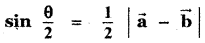(ii)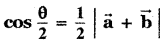(iii)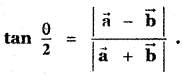Given $$\vec{a}$$ and $$\vec{b}$$ are unit vectors.
∴ |$$\vec{a}$$| = 1 and |$$\vec{b}$$| = 1

(i)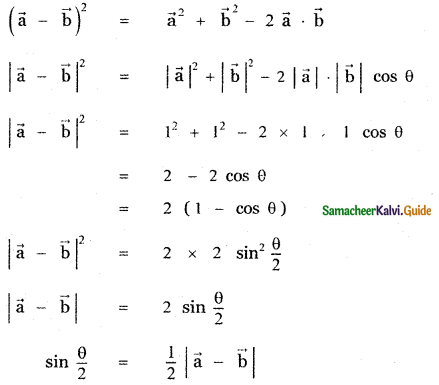(ii)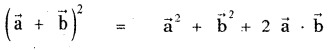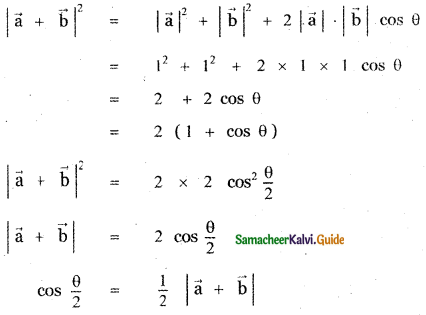(iii)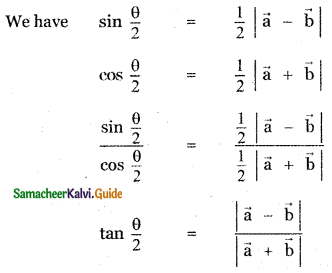Question 11.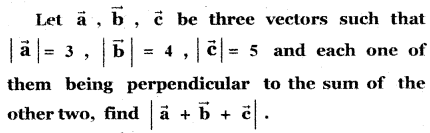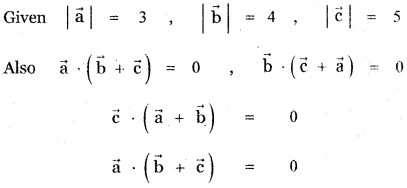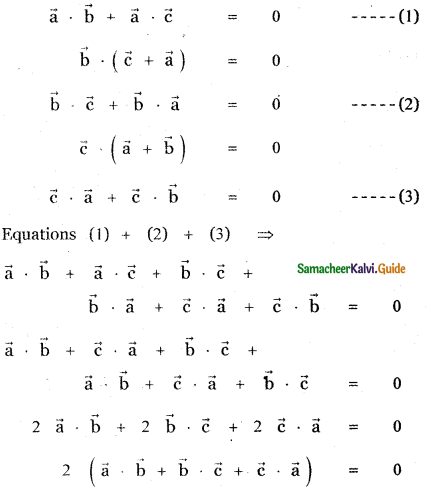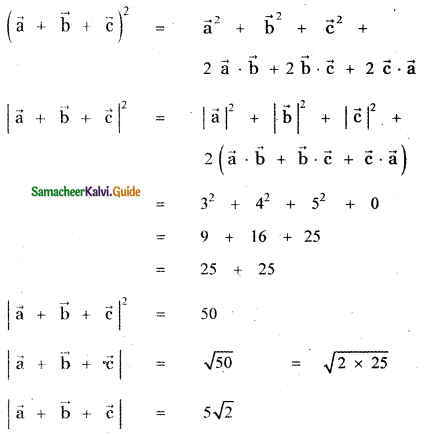Question 12.
Find the projection of the vector î + 3ĵ + 7k̂ on the vector 2î + 6ĵ + 3k̂
The given vectors are î + 3ĵ + 7k̂ and 2î + 6ĵ + 3k̂
Projection of î + 3ĵ + 7k̂ on 2î + 6ĵ + 3k̂ is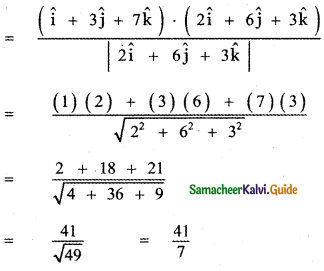Question 13.
Find λ, when the projection $$\vec{a}$$ = λî + ĵ + 4k̂ on $$\vec{b}$$ = 2î + 6ĵ + 3k̂ is 4 units.
$$\vec{a}$$ = λî + ĵ + 4k̂ , $$\vec{b}$$ = 2î + 6ĵ + 3k̂
Also given that projection of $$\vec{a}$$ and $$\vec{b}$$ is 4 units.